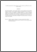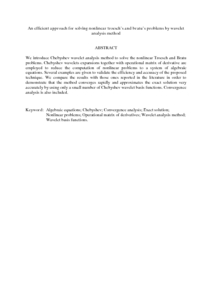# An efficient approach for solving nonlinear troesch's and bratu's problems by wavelet analysis method

## Citation

Nasab, A. Kazemi and Atabakan, Z. Pashazadeh and Kilicman, Adem (2013) An efficient approach for solving nonlinear troesch's and bratu's problems by wavelet analysis method. Mathematical Problems in Engineering, 2013. art. no. 825817. pp. 1-10. ISSN 1024-123X

## Abstract

We introduce Chebyshev wavelet analysis method to solve the nonlinear Troesch and Bratu problems. Chebyshev wavelets expansions together with operational matrix of derivative are employed to reduce the computation of nonlinear problems to a system of algebraic equations. Several examples are given to validate the efficiency and accuracy of the proposed technique. We compare the results with those ones reported in the literature in order to demonstrate that the method converges rapidly and approximates the exact solution very accurately by using only a small number of Chebyshev wavelet basis functions. Convergence analysis is also included.Preview
PDF (Abstract)
An efficient approach for solving nonlinear troesch.pdfView Item